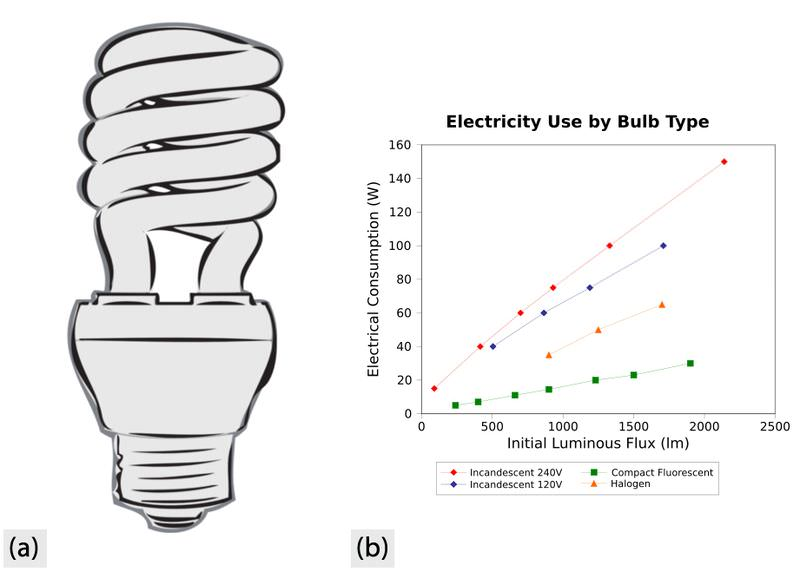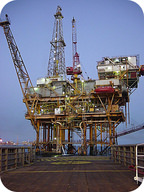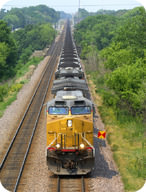# 20.2: Obtaining Energy Resources

•• Contributed by CK12
• CK12Have you converted to compact fluorescent light bulbs at your house?

Compact fluorescent light bulbs are more efficient than incandescent light bulbs. Look at the chart and try to see how much more efficient. The answer is that they could be as much as six times more efficient. So why aren't all people using compact fluorescent bulbs all the time? Early ones were large and expensive, and many people don't like the color of the light. But they are much more environmentally friendly.

## Net Energy

Net energy is the amount of useable energy available from a resource after subtracting the amount of energy needed to make the energy from that resource available. For example, every 5 barrels of oil that are made available for use require 1 barrel for extracting and refining the petroleum. What is the net energy from this process? About 4 barrels (5 barrels minus 1 barrel).

What happens if the energy needed to extract and refine oil increases? Why might that happen? The energy cost of an energy resource increases when the easy deposits of that resource have already been consumed. For example, if all the nearshore petroleum in a region has been extracted, more costly drilling must take place further offshore (Figure below). If the energy cost of obtaining energy increases, the resource will be used even faster.Offshore drilling is taking place in deeper water than before. It takes a lot of energy to build a deep drilling platform and to run it.

### Net-Energy Ratio

The net-energy ratio demonstrates the difference between the amount of energy available in a resource and the amount of energy used to get it. If it takes 8 units of energy to make available 10 units of energy, then the net-energy ratio is 10/8 or 1.25. What does a net-energy ratio larger than 1 mean? What if the net-energy ratio is less than 1? A net-energy ratio larger than 1 means that there is a net gain in usable energy; a net-energy ratio smaller than one means there is an overall energy loss.

Table below shows the net-energy ratios for some common energy sources.

Net-Energy Ratios for Common Energy Sources
Energy Source Net-energy Ratio
Solar Energy 5.8
Natural Gas 4.9
Petroleum 4.5
Coal-fired Electricity 2.5-5.1

Notice from the table that solar energy yields much more net energy than other sources. This is because it takes very little energy to get usable solar energy. Sunshine is abundant and does not need to be found, extracted, or transported very far. The range for coal-fired electricity is because of the differing costs of transporting the coal. What does this suggest about using coal to generate electricity? The efficiency is greater in areas where the coal is locally mined and does not have to be transported great distances (Figure below).Obtaining coal for energy takes a lot of energy. The coal must be located, extracted, refined, and transported.

This is not to say that solar energy is less expensive than other types of energy. The cost of energy is dependent on lots of different factors, such as the cost of the equipment needed to harness the energy. If solar power cost less to use, it would be more widespread.

### Energy Efficiency

Energy efficiency describes how much useful work is extracted from one unit of energy. Remember that although energy is not created or destroyed, it's just transferred from one form to another, some energy is nearly always lost in the transfer as heat. By saying that the work must be useful, it is possible to account for the energy that is lost to non-useful work. For example, some energy may not be doing useful work if the equipment is not running well (maybe a piston is moving sideways a bit rather than just up and down).

Higher energy efficiency is desirable because:

• Less energy is being wasted.
• Non-renewable resources will last longer.
• The cost is kept lower.

Because so much of the energy we use is from fossil fuels, we need to be especially concerned about using them efficiently. Sometimes our choices affect energy efficiency. For example, transportation by cars and airplanes is less energy-efficient than transportation by boats and trains.

## Summary

• Net energy is the amount of that is actually useable from an energy resource. Net-energy ratio is the ratio of the amount of useable energy from a resource and the amount it takes to make that energy useful.
• Many factors besides net-energy ratio go into determining if a type of energy will be used.
• An energy source with high energy efficiency provides a lot of work for the amount of energy used.

## Review

1. Compare and contrast net energy, the net-energy ratio, and energy efficiency.
2. Since the net-energy ratio for solar energy is higher than other types of energy, why don't we use solar for electricity almost exclusively?
3. Why would the energy needed to make a type of energy useful increase or decrease? In other words, why would the net-energy ratio change?

## Explore More

Use this resource to answer the questions that follow.

1. Besides energy, what is lost when energy drips away?
2. Why should you replace incandescent bulbs with CFLS?
3. Why is it good to plug electronics into a power strip?
4. Why should you use a programmable thermostat?
5. What is the purpose of insulation?
6. Why is an old appliance an energy sink?
7. If you did these things, how much money would you save in a decade on average (in Minnesota)?